## Exercise 12: Estimating speciation & extinction rates from phylogenies

In this tutorial, we will use ape & diversitree to estimate diversification rates from phylogenies.

### 1. Estimating the speciation & extinction rates from the phylogeny of darters using ape

To start with, let's use our darter tree of Near et al. (2011) that we examined in the previous exercise.

``````library(phytools)
``````

``````darter.tree<-read.tree("etheostoma_percina_chrono.tre")
``````

The Nee et al. method for estimating speciation & extinction rates from a completely sampled phylogeny is implemented in the ape function `birthdeath`. Let's fit this model to our tree:

``````fit.bd<-birthdeath(darter.tree)
``````
``````## Warning in nlm(function(p) dev(p, p), c(0.1, 0.2), hessian = TRUE):
## NA/Inf replaced by maximum positive value

## Warning in nlm(function(p) dev(p, p), c(0.1, 0.2), hessian = TRUE):
## NA/Inf replaced by maximum positive value

## Warning in nlm(function(p) dev(p, p), c(0.1, 0.2), hessian = TRUE):
## NA/Inf replaced by maximum positive value

## Warning in nlm(function(p) dev(p, p), c(0.1, 0.2), hessian = TRUE):
## NA/Inf replaced by maximum positive value

## Warning in nlm(function(p) dev(p, p), c(0.1, 0.2), hessian = TRUE):
## NA/Inf replaced by maximum positive value

## Warning in nlm(function(p) dev(p, p), c(0.1, 0.2), hessian = TRUE):
## NA/Inf replaced by maximum positive value

## Warning in nlm(function(p) dev(p, p), c(0.1, 0.2), hessian = TRUE):
## NA/Inf replaced by maximum positive value

## Warning in nlm(function(p) dev(p, p), c(0.1, 0.2), hessian = TRUE):
## NA/Inf replaced by maximum positive value
``````
``````## Error in while (bar() < Dev + 3.84) p <- p + s * i: missing value where TRUE/FALSE needed
``````

We get errors because our tree is incompletely resolved. Let's resolve the tree using `multi2di` and tree again:

``````darter.tree<-multi2di(darter.tree)
fit.bd<-birthdeath(darter.tree)
fit.bd
``````
``````##
## Estimation of Speciation and Extinction Rates
##             with Birth-Death Models
##
##      Phylogenetic tree: darter.tree
##         Number of tips: 201
##               Deviance: -741.7514
##         Log-likelihood: 370.8757
##    Parameter estimates:
##       d / b = 0.06330651   StdErr = 0.1640933
##       b - d = 0.2212409   StdErr = 0.02342253
##    (b: speciation rate, d: extinction rate)
##    Profile likelihood 95% confidence intervals:
##       d / b: [-0.1838519, 0.2583648]
##       b - d: [0.1914747, 0.2539717]
``````

For computational reasons, `birthdeath` fits a re-parameterization of the birth-death model - estimating the derived parameters `b-d` and `b/d`. To solve for `b` and `d`, we can use this custom function on our fitted model object:

``````bd<-function(x){
if(class(x)!="birthdeath") stop("x should be an object of class 'birthdeath'")
b<-x\$para/(1-x\$para)
d<-b-x\$para
setNames(c(b,d),c("b","d"))
}
bd(fit.bd)
``````
``````##          b          d
## 0.23619344 0.01495258
``````

### 2. Using simulation to explore birth-death trees in phytools

Now that we have seen one empirical case, let's use phytools to explore the properties of birth-death trees (trees that arise by stochastic, constant-rate speciation & extinction).

First, simulate birth & death trees in phytools. phytools function `pbtree` (even though originally a pure-birth tree simulator only) can simulate under a variety of different models.

Let's simulate under pure-birth using phytools. One experiment that might be fun is to examine the variance in number of lineages among trees given a particular set of simulation conditions:

``````set.seed(10)
b<-(log(100)-log(2))/100 ## results in E(lineages)=100 after t=100
b
``````
``````##  0.03912023
``````
``````trees<-pbtree(b=b,t=100,nsim=200)
n<-sapply(trees,Ntip)
mean(n) ## should be 100
``````
``````##  104.72
``````
``````var(n) ## will tend to be very large
``````
``````##  5450.816
``````
``````hist(n,xlab="number of species",main="number of species",col="grey",breaks=20)
lines(rep(mean(n),2),par()\$usr[3:4],lty="dashed",lwd=2)
``````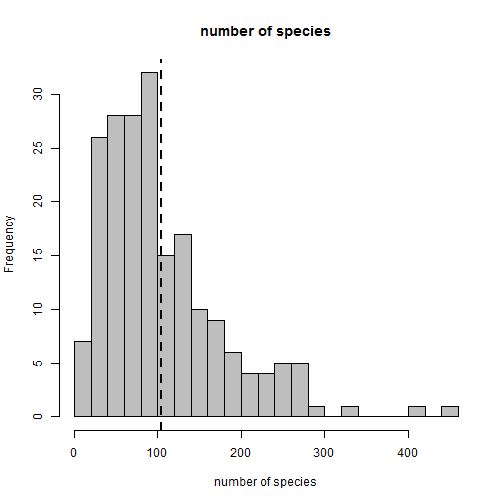From this we can see that the variance under pure-birth can be great. This increases when there is also extinction. For instance:

``````d<-0.01
dtrees<-pbtree(b=b+d,d=d,t=100,nsim=200,extant.only=TRUE,quiet=TRUE)
n<-sapply(dtrees,function(x) if(!is.null(x)) Ntip(x) else 0)
mean(n) ## should be 100
``````
``````##  96.03
``````
``````var(n) ## will tend to be very large
``````
``````##  6062.069
``````
``````hist(n,xlab="number of species",main="number of species",col="grey",breaks=20)
lines(rep(mean(n),2),par()\$usr[3:4],lty="dashed",lwd=2)
``````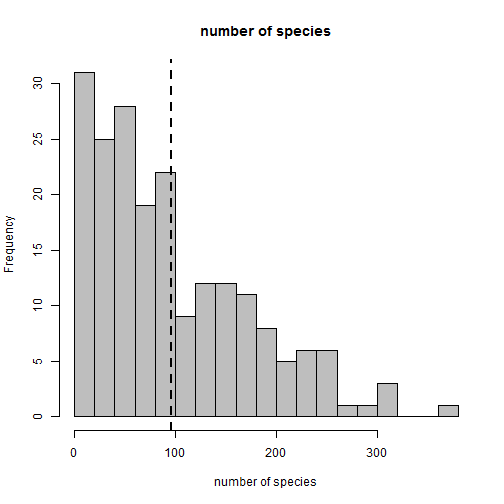So we can see that the expected number of tips in a tree is determined only by `b - d`; however the variance increases with `d`!

### 3. Statistical properties of the Nee et al. method

Although some recent papers have criticized the Nee et al. method (and others defended it), the point should still be made that when the model assumptions hold, it does perform just as it is supposed to.

Specifically, we can demonstrate by fitting the model over our simulated distribution of trees, that the birth & death rates are unbiasedly estimated (albeit with substantial variance).

I'm going to start by removing all the trees from our sample that either went extinct (only in the birth-death trees) or left fewer than 10 extant species (we would never fit this model for such a small tree).

``````## remove any trees with no taxa
trees<-trees[!sapply(trees,is.null)]
## remove any trees with <10 taxa
trees<-trees[sapply(trees,function(x) length(x\$tip.label)>=10)]
obj<-lapply(trees,birthdeath)
``````
``````## Warning in sqrt(diag(inv.hessian)): NaNs produced

## Warning in sqrt(diag(inv.hessian)): NaNs produced

## Warning in sqrt(diag(inv.hessian)): NaNs produced

## Warning in sqrt(diag(inv.hessian)): NaNs produced
``````
``````BD<-t(sapply(obj,bd))
par(mfrow=c(2,1))
hh<-hist(BD[,1],breaks=20,xlab="estimated birth rate",main="birth rate")
lines(rep(mean(BD[,1]),2),c(0,max(hh\$counts)),lty="dotted",lwd=2,col="red")
hh<-hist(BD[,2],breaks=20,xlab="estimated death rate",main="death rate")
lines(rep(mean(BD[,2]),2),c(0,max(hh\$counts)),lty="dotted",lwd=2,col="red")
``````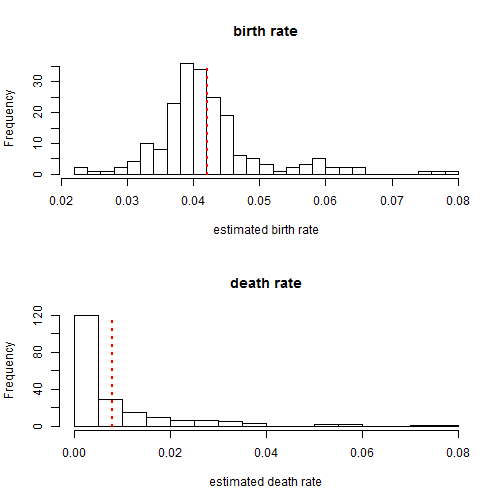Now let's try the same thing, but with our birth-death rate:

``````dtrees<-dtrees[!sapply(dtrees,is.null)]
dtrees<-dtrees[sapply(dtrees,function(x) length(x\$tip.label)>=10)]
obj<-lapply(trees,birthdeath)
``````
``````## Warning in sqrt(diag(inv.hessian)): NaNs produced

## Warning in sqrt(diag(inv.hessian)): NaNs produced

## Warning in sqrt(diag(inv.hessian)): NaNs produced

## Warning in sqrt(diag(inv.hessian)): NaNs produced
``````
``````BD<-t(sapply(obj,bd))
colMeans(BD)
``````
``````##           b           d
## 0.042108981 0.008014036
``````
``````par(mfrow=c(2,1))
hh<-hist(BD[,1],breaks=20,xlab="estimated birth rate",main="birth rate")
lines(rep(mean(BD[,1]),2),c(0,max(hh\$counts)),lty="dotted",lwd=2,col="red")
hh<-hist(BD[,2],breaks=20,xlab="estimated death rate",main="death rate")
lines(rep(mean(BD[,2]),2),c(0,max(hh\$counts)),lty="dotted",lwd=2,col="red")
``````So, we can see that Nee's method allows us to estimate diversification rates from reconstructed birth-death phylogenies; however these parameters are sometimes estimated with wide confidence limits, something that we can understand best by considering the very broad variance in outcome from the generating process.

### 4. Fitting a birth-death model using diversitree

diversitree, by Rich Fitzjohn, is a very powerful R package for studying diversification processes on phylogenies. We will be using diversitree in further exercises; however, in this exercise, we will essentially repeat some analyses we have already conducted with ape and phytools, but with the goal of learning some of the basic functionality of the diversitree packages.

``````library(diversitree)
``````

Now, we can fit a Yule (pure-birth) model using diversitree. The way that the functions of diversitree work, we first built a model - then we use a generic function to optimize it. One of the advantages of diversitree is that it allows us to use incompletely sampled trees, and take that explicitly into consideration when fitting the model. In this case, we know the darter tree to contain about 201 of 216 of described species, more or less 93%.

``````pbModel<-make.yule(darter.tree,sampling.f=201/216)
pbMLFit<-find.mle(pbModel,x.init=0.1)
pbMLFit
``````
``````## \$par
##    lambda
## 0.2374681
##
## \$lnLik
##  370.7178
##
## \$counts
##  7
##
## \$code
##  1
##
##  -0.0003498712
##
## \$method
##  "nlm"
##
## \$func.class
##  "yule"     "bd"       "dtlik"    "function"
##
## attr(,"func")
## Yule (pure birth) likelihood function:
##   * Parameter vector takes 1 elements:
##      - lambda
##   * Function takes arguments (with defaults)
##      - pars: Parameter vector
##      - condition.surv [TRUE]: Condition likelihood on survial?
##   * Phylogeny with 201 tips and 200 nodes
##      - Taxa: Percidae_Etheostoma_cinereum_Near2011, ...
##   * Reference:
##      - Nee et al. (1994) doi:10.1098/rstb.1994.0068
## R definition:
## function (pars, condition.surv = TRUE)
## attr(,"class")
##  "fit.mle.bd" "fit.mle"
``````

Of course, we can also fit a birth-death model. Let's do that:

``````bdModel<-make.bd(darter.tree,sampling.f=201/216)
bdMLFit<-find.mle(bdModel,c(0.1,0.05),method = "optim",lower = 0)
bdMLFit
``````
``````## \$par
##     lambda         mu
## 0.25381855 0.03257651
##
## \$lnLik
##  370.8757
##
## \$counts
##       13       13
##
## \$convergence
##  0
##
## \$message
##  "CONVERGENCE: REL_REDUCTION_OF_F <= FACTR*EPSMCH"
##
## \$optim.method
##  "L-BFGS-B"
##
## \$method
##  "optim"
##
## \$func.class
##  "bd"       "dtlik"    "function"
##
## attr(,"func")
## Constant rate birth-death likelihood function:
##   * Parameter vector takes 2 elements:
##      - lambda, mu
##   * Function takes arguments (with defaults)
##      - pars: Parameter vector
##      - condition.surv [TRUE]: Condition likelihood on survial?
##      - intermediates [FALSE]: Also return intermediate values?
##   * Phylogeny with 201 tips and 200 nodes
##      - Taxa: Percidae_Etheostoma_cinereum_Near2011, ...
##   * Reference:
##      - Nee et al. (1994) doi:10.1098/rstb.1994.0068
## R definition:
## function (pars, condition.surv = TRUE, intermediates = FALSE)
## attr(,"class")
##  "fit.mle.bd" "fit.mle"
``````

Again, using diversitree it is easy to compare the two models. This is essentially a test for the signature of extinction in our tree.

``````# compare models
anova(bdMLFit, pure.birth = pbMLFit)
``````
``````##            Df  lnLik     AIC   ChiSq Pr(>|Chi|)
## full        2 370.88 -737.75
## pure.birth  1 370.72 -739.44 0.31567     0.5742
``````

Here we see that although our MLE for the extinction rate is non-zero, the model does not significantly increase fit.

Let's visualize the likelihood surface for λ & μ to see how broad it is for the extinction rate, μ.

``````b<-seq(0.1,10,by=0.1)*bdMLFit\$par
d<-b
L<-sapply(b,function(b,d) sapply(d,function(d,b) bdModel(c(b,d)),b=b),d=d)
persp(b,d,L,phi=45,theta=-30)
``````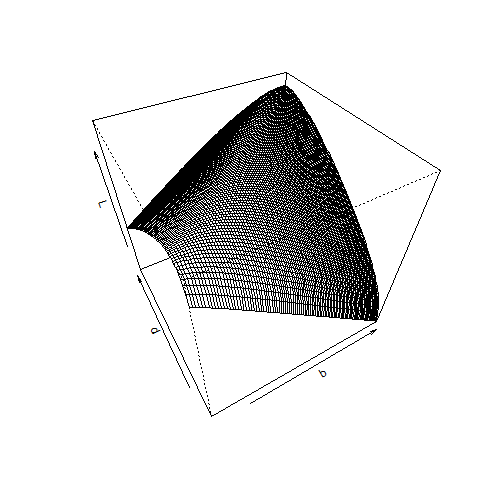``````contour(b,d,L,nlevels=50)
``````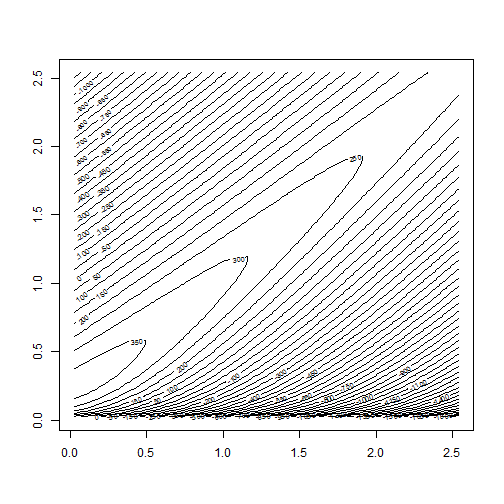``````filled.contour(b,d,L,nlevels=50,color.palette=heat.colors)
``````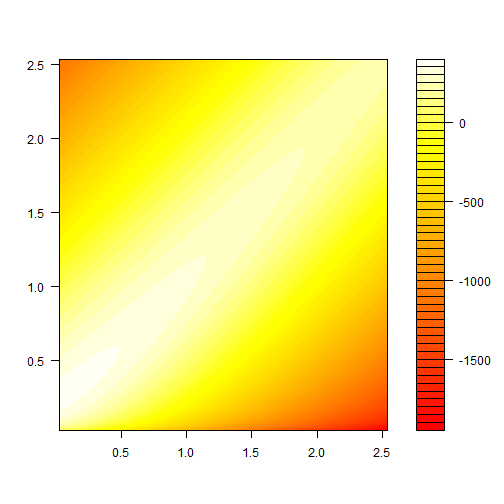You can also try:

``````library(rgl)
persp3d(b,d,L)
``````

Finally, diversitree also very easily permits us to design and run a Bayesian MCMC analysis of diversification. We do that as follows:

``````bdSamples <- mcmc(bdModel, bdMLFit\$par, nsteps = 1e+05, lower = c(0, 0),
upper = c(Inf,Inf), w = c(0.1, 0.1), fail.value = -Inf,
print.every = 10000)
``````
``````## 10000: {0.2621, 0.0153} -> 370.33622
## 20000: {0.2858, 0.1135} -> 369.73068
## 30000: {0.2337, 0.0351} -> 370.04706
## 40000: {0.2916, 0.0604} -> 369.94619
## 50000: {0.2587, 0.0591} -> 370.63810
## 60000: {0.2155, 0.0016} -> 369.72629
## 70000: {0.3046, 0.1091} -> 369.96390
## 80000: {0.2711, 0.0926} -> 370.00157
## 90000: {0.2677, 0.0563} -> 370.79250
## 100000: {0.2433, 0.0578} -> 369.80152
``````
``````## visualize the posterior sample
postSamples <- bdSamples[c("lambda", "mu")]
profiles.plot(postSamples, col.line = c("red", "blue"), las = 1, legend = "topright")
``````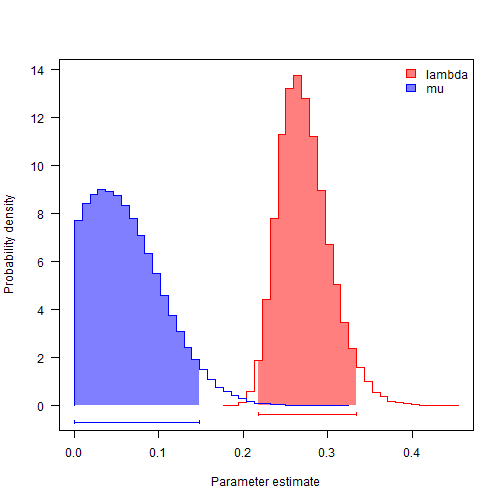``````# often estimates of r (= lambda-mu) are more precise than either lambda and
# mu
postSamples\$r <- with(bdSamples, lambda - mu)
postSamples\$eps <- with(bdSamples, mu/lambda)
profiles.plot(postSamples[, c("r", "eps")], col.line = c("red", "blue"), las = 1,
legend = "topright")
``````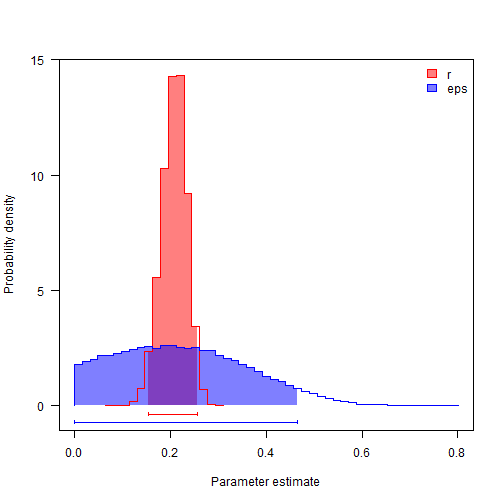### Challenge Problem 8: Estimating diversification rates

Download the following file containing 100 trees (from a Bayesian posterior sample) of elopomorph eels. Only about 20% of extant eels are represented in these data!

Use diversitree to estimate the speciation and extinction rate for each tree. What is the average speciation and extinction rate across all trees? In what terms are these rates measured? What is the variance and speciation & extinction rates among trees?

Written by Liam J. Revell & Luke J. Harmon. Last updated 1 Jul. 2015